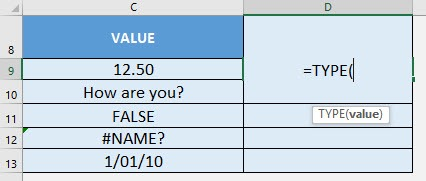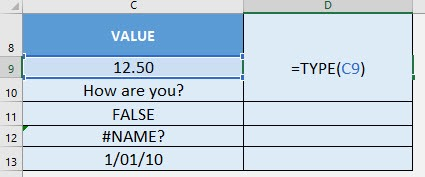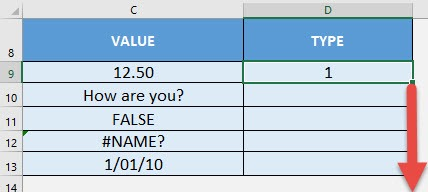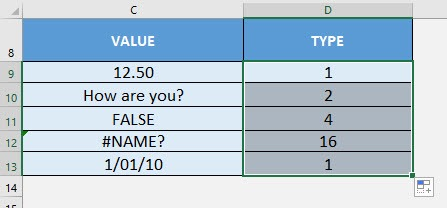What does it do?

Gets the data type from a value

Formula breakdown:

=TYPE(value)

What it means:

=TYPE(value to be checked for its data type)

Do you need to check the data type of your values? The TYPE Formula in Excel is perfect for this!

The TYPE- Formula checks for the following data types:

• 1 – number
• 2 – text
• 4 – logical value
• 16 – error value
• 64 – array

I explain how you can do this below:STEP 1: We need to enter the TYPE function in a blank cell:

## =TYPE(STEP 2: The TYPE arguments:

## value

What is the value to get the data type from?

Select the cell containing the value:

## =TYPE(C9)Apply the same formula to the rest of the cells by dragging the lower right corner downwards.You now have your data types!How to Use the TYPE Formula in Excel